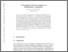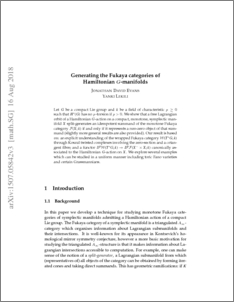# Generating the Fukaya categories of Hamiltonian G-manifolds

Evans, Jonathan David and Lekili, YankI (2019) Generating the Fukaya categories of Hamiltonian G-manifolds. Journal of the American Mathematical Society, 32 (1). pp. 119-162. ISSN 1088-6834Preview
PDF (1507.05842)
1507.05842.pdf - Accepted Version

## Abstract

Abstract: Let $G$ be a compact Lie group, and let $k$ be a field of characteristic $p \geq 0$ such that $H^*(G)$ has no $p$-torsion if $p>0$. We show that a free Lagrangian orbit of a Hamiltonian $G$-action on a compact, monotone, symplectic manifold $X$ split-generates an idempotent summand of the monotone Fukaya category $\mathcal {F}(X; k)$ if and only if it represents a nonzero object of that summand (slightly more general results are also provided). Our result is based on an explicit understanding of the wrapped Fukaya category $\mathcal {W}(T^*G; k)$ through Koszul twisted complexes involving the zero-section and a cotangent fibre and on a functor $D^b \mathcal {W}(T^*G; k) \to D^b\mathcal {F}(X^{-} \times X; k)$ canonically associated to the Hamiltonian $G$-action on $X$. We explore several examples which can be studied in a uniform manner, including toric Fano varieties and certain Grassmannians.

Item Type:
Journal Article
Journal or Publication Title:
Journal of the American Mathematical Society
Uncontrolled Keywords:
/dk/atira/pure/subjectarea/asjc/2600
Subjects:
Departments:
ID Code:
132429
Deposited By:
Deposited On:
02 Apr 2019 13:40
Refereed?:
Yes
Published?:
Published